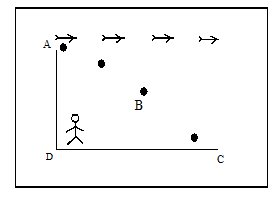An object is dropped from an aeroplane which is moving horizontally when it is at point A, then among the following, the correct statement is1) The path of the object as seen by the pilot is vertically downwards2) The path of the object as seen by the observer on the ground is the curve ABC3) The path of the object as seen by the observer on the ground is AD4) The path of the object as seen by the pilot is ABC

# An object is dropped from an aeroplane which is moving horizontally when it is at point A, then among the following, the correct statement is1) The path of the object as seen by the pilot is vertically downwards2) The path of the object as seen by the observer on the ground is the curve ABC3) The path of the object as seen by the observer on the ground is AD4) The path of the object as seen by the pilot is ABC

1. A

1,2 and 3 are correct

2. B

1,2 and 4 are correct

3. C

1 and 2 are correct

4. D

2 and 3 are correct

Register to Get Free Mock Test and Study Material

+91

Verify OTP Code (required)

### Solution:

By conceptualRegister to Get Free Mock Test and Study Material

+91

Verify OTP Code (required)